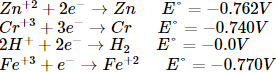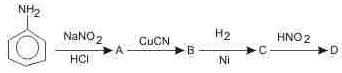Courses

SRMJEEE Chemistry Mock Test - 9

35 Questions MCQ Test SRMJEEE Subject Wise & Full Length Mock Tests | SRMJEEE Chemistry Mock Test - 9

Description
Attempt SRMJEEE Chemistry Mock Test - 9 | 35 questions in 50 minutes | Mock test for JEE preparation | Free important questions MCQ to study SRMJEEE Subject Wise & Full Length Mock Tests for JEE Exam | Download free PDF with solutions
QUESTION: 1

Solution:
QUESTION: 2

Solution:
QUESTION: 3

Which of the following element is responsible for oxidation of water to O₂ in biological processes?

Solution:
QUESTION: 4

Which one of the following has the minimum boiling point?

Solution:
QUESTION: 5

The compound formed in the borax bead test of Cu2⁺ ion in oxidising flame is

Solution:
QUESTION: 6

The reacting ion in the nitration of benzene is

Solution:
QUESTION: 7

Our sun belongs to which of the following spectra?

Solution:
QUESTION: 8

The most stable carbonium ion is:

Solution:
QUESTION: 9

Consider the following compounds :
1. CH₂N₂
2. CH₂ = C = O
3. CH₂I₂
Those compounds which would generate a carbene on exposure to ultraviolet light include :

Solution:
QUESTION: 10

Which of the following molecules has the highest dipole moment?

Solution:
QUESTION: 11

Which of the following bonds has highest average bond energy ?

Solution:
QUESTION: 12

The pieces of wood burn faster than a log of wood of the same mass, because

Solution:
QUESTION: 13

The reaction N₂ + O₂ → 2NO is endothermic . The forward reaction is

Solution:
QUESTION: 14

The reaction of chloroform with alcoholic KOH and p-toludine forms

Solution:
QUESTION: 15

An exothermic reaction is one in which the reacting substances

Solution:
QUESTION: 16

Substances which bring body temperature down are known as

Solution:
QUESTION: 17

In which of the following compounds,carbon uses sp3 hybrid orbitals only for bonding ?

Solution:
QUESTION: 18

The standard reduction potential at 298 K for the following half cell reaction are given belowWhich one is the strongest reducing agent?

Solution:
QUESTION: 19

Which of the following is correctly matched?

Solution:

C-Cl bond length is 0.176 nm; ionic radii of Na+ and F - are 0.095 and 0.136 nm respectively; C-C bond length is 0.154 nm.

QUESTION: 20

At what temperature will the average speed of CH₄ molecules have the same value as O₂ has at 300 K

Solution:
QUESTION: 21

Select the true statement about benzene from amongst the following

Solution:
QUESTION: 22

The pH indicators are

Solution:
QUESTION: 23

Which of the following is not isomeric with diethyl ether?

Solution:
QUESTION: 24

A human body required 0.01 μC activity of radioactive substance for 24 hrs. Half life of radioactive substance is 6 hours. Then injection of maximum activity of radioactive substance (in μC) can be injected is

Solution:
QUESTION: 25

Aniline in a set of reactions yielded a product DThe structure of D is

Solution:
QUESTION: 26

Dow's reaction involves

Solution:
QUESTION: 27

Select incorrect statement:

Solution:
QUESTION: 28

In a polymer 30% molecules have molecular weight 20,000, 40% molecules have molecular weight 30,000 and the rest 30% molecules have molecular weight 60000. The weight average molecular weight of the polymer is

Solution:
QUESTION: 29

In paper chromotagraphy,

Solution:
QUESTION: 30

The oxidation number of Mn in KMnO₄ is

Solution:
QUESTION: 31

Semiconductors are derived from compounds of

Solution:
QUESTION: 32

Which acetone and chloroform are mixed, hydrogen bonding takes place between them. Such a liquid pair will cause

Solution:
QUESTION: 33

In a flask of volume V litres, 0.2 mol of oxygen, 0.4 mol of nitrogen, 0.1 mole of NH₃ and 0.3 mol of He are enclosed at 27°C. If the total pressure exerted by these non reacting gases is one atmosphere, the partial pressure exerted by nitrogen is

Solution:
QUESTION: 34

How much quick lime can be obtained from 25 gm of CaCO₃ ?

Solution:
QUESTION: 35

The reactions in zeolite catalysts depend upon

Solution:Use Code STAYHOME200 and get INR 200 additional OFF Use Coupon Code

Track your progress, build streaks, highlight & save important lessons and more!

Similar ContentRelated tests